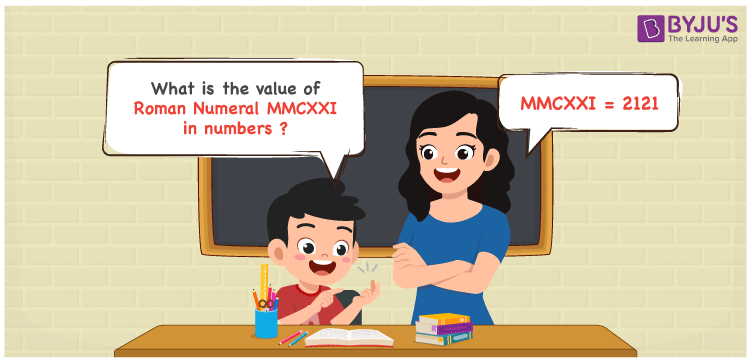Checkout JEE MAINS 2022 Question Paper Analysis : Checkout JEE MAINS 2022 Question Paper Analysis :

# MMCXXI Roman Numerals

MMCXXI Roman Numerals is 2121. In this article, expanding MMCXXI to numbers is explained in brief based on the understanding abilities of students. If the conversion steps are learnt well, most of the questions based on these concepts can be answered with ease. Roman numerals articles have all the required information to enhance the analytical and logical thinking skills among students. Therefore, MMCXXI can be indicated as 2121 in numbers.

 Number Roman Numeral 2121 MMCXXI## How to Write MMCXXI Roman Numerals in Numbers?

The steps used to mention MMCXXI in numeric form can be learned by referring to the expansion given.

MMCXXI = M + M + C + X + X + 1

MMCXXI = 1000 + 1000 + 100 + 10 + 10 + 1

MMCXXI = 2121

## Video Lesson on Roman Numerals## Frequently Asked Questions on MMCXXI Roman Numerals

### Why is the roman numeral of 2121 written as MMCXXI?

We know that

MMCXXI = M + M + C + X + X + 1

MMCXXI = 1000 + 1000 + 100 + 10 + 10 + 1

MMCXXI = 2121

### Determine the value of 2000 + 121.

We know that

2000 + 121 = 2121

Hence, the value of 2000 + 121 is 2121 which is written as MMCXXI.

### What is the remainder we obtain when MMCXXI is divided by XI?

We know that

MMCXXI = 2121

XI = 11

So the remainder we obtain when MMCXXI is divided by XI is IX.